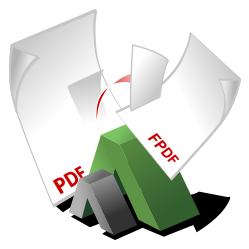# FPDI

## Simple Introduction Demo of FPDI

This demo simply imports a single page of an existing PDF document and adds text in front of it.

PHP
```<?php

require_once('fpdf/fpdf.php');

// initiate FPDI
\$pdf = new \setasign\Fpdi\Fpdi();
// set the source file
\$pdf->setSourceFile('PdfDocument.pdf');
// import page 1
\$tplIdx = \$pdf->importPage(1);
// use the imported page and place it at position 10,10 with a width of 100 mm
\$pdf->useTemplate(\$tplIdx, 10, 10, 100);

// now write some text above the imported page
\$pdf->SetFont('Helvetica');
\$pdf->SetTextColor(255, 0, 0);
\$pdf->SetXY(30, 30);
\$pdf->Write(0, 'This is just a simple text');

\$pdf->Output('I', 'generated.pdf');
```